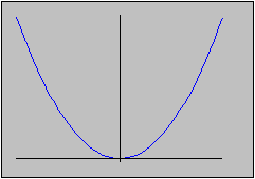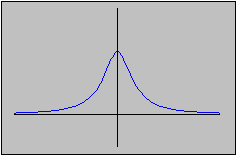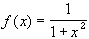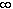#Interactive Real Analysis

Next | Previous | Glossary | Map

## 6.4. Topology and Continuity

### Examples 6.4.3:

Is it true that if f is continuous, then the image of an open set is again open ? How about the image of a closed set ?

This is true for inverse images but not for images. Consider the example of a parabola, which certainly represents a continuous function:f(x) = x2
Then the image of the set (-1, 1) is the set [0, 1). That set is neither open nor closed; in particular, it is not open.

To find a counterexample for images of closed sets, let's look at the following function:This function is continuous on the whole real line, and the image of the set [0,) is the set (0, 1]. Therefore we have found a closed set whose image under a continuous function is not closed (nor open).
Next | Previous | Glossary | Map